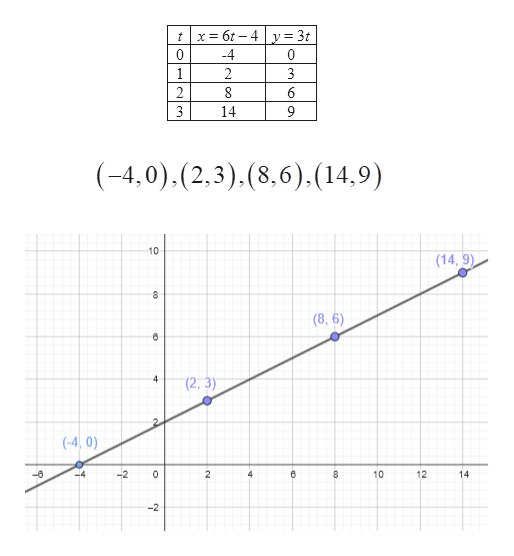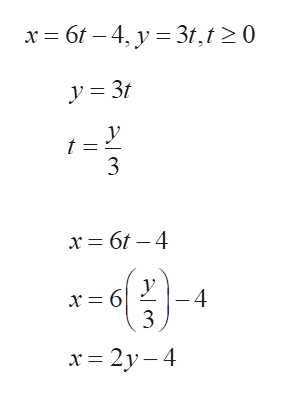# The question says:"A pair of paraetric equations is given. a.) Sketch the curve represented by the parametric equations. b.) Find a rectangular-coordinate equation for the curve by eliminating the parameter."x=6t-4, y=3t, t>=0

Question
1 views

The question says:

"A pair of paraetric equations is given. a.) Sketch the curve represented by the parametric equations. b.) Find a rectangular-coordinate equation for the curve by eliminating the parameter."

x=6t-4, y=3t, t>=0

check_circle

Step 1

To sketch the graph, the points (x, y) are to be determined for different t values and then the points are be plotted and joined.

The table of value for x and y is given by:

Hence the points are given by

Plotting the points:

From the points it is seen that they are on a straight line.help_outlineImage Transcriptionclosetx6t-4y3t 0 4 0 1 2 3 2 8 6 3 14 9 (-4,0).(2,3).(8,6),(14,9) 10 (14,9) 8 (8, 6) 4 (2,3) (-4, 0) -2 0 2 10 12 -6 4 4 14 -2 st. fullscreen
Step 2

b.

The rectangular coordinate can be determined by eliminating t.

Given parametric equations are:

...help_outlineImage Transcriptionclosex 6t -4, y 3,t 0 3 y -4 x = 6 x 2y-4 fullscreen

### Want to see the full answer?

See Solution

#### Want to see this answer and more?

Solutions are written by subject experts who are available 24/7. Questions are typically answered within 1 hour.*

See Solution
*Response times may vary by subject and question.
Tagged in

### Calculus